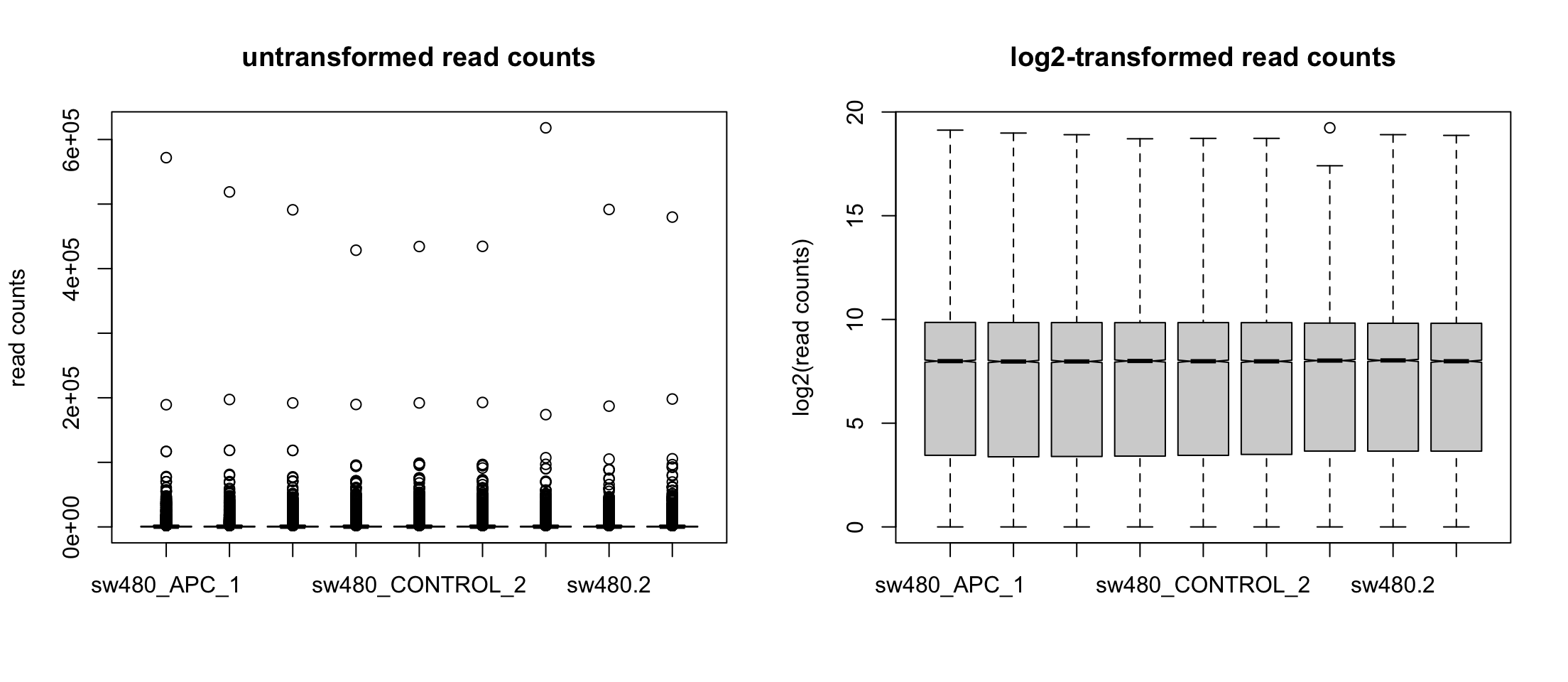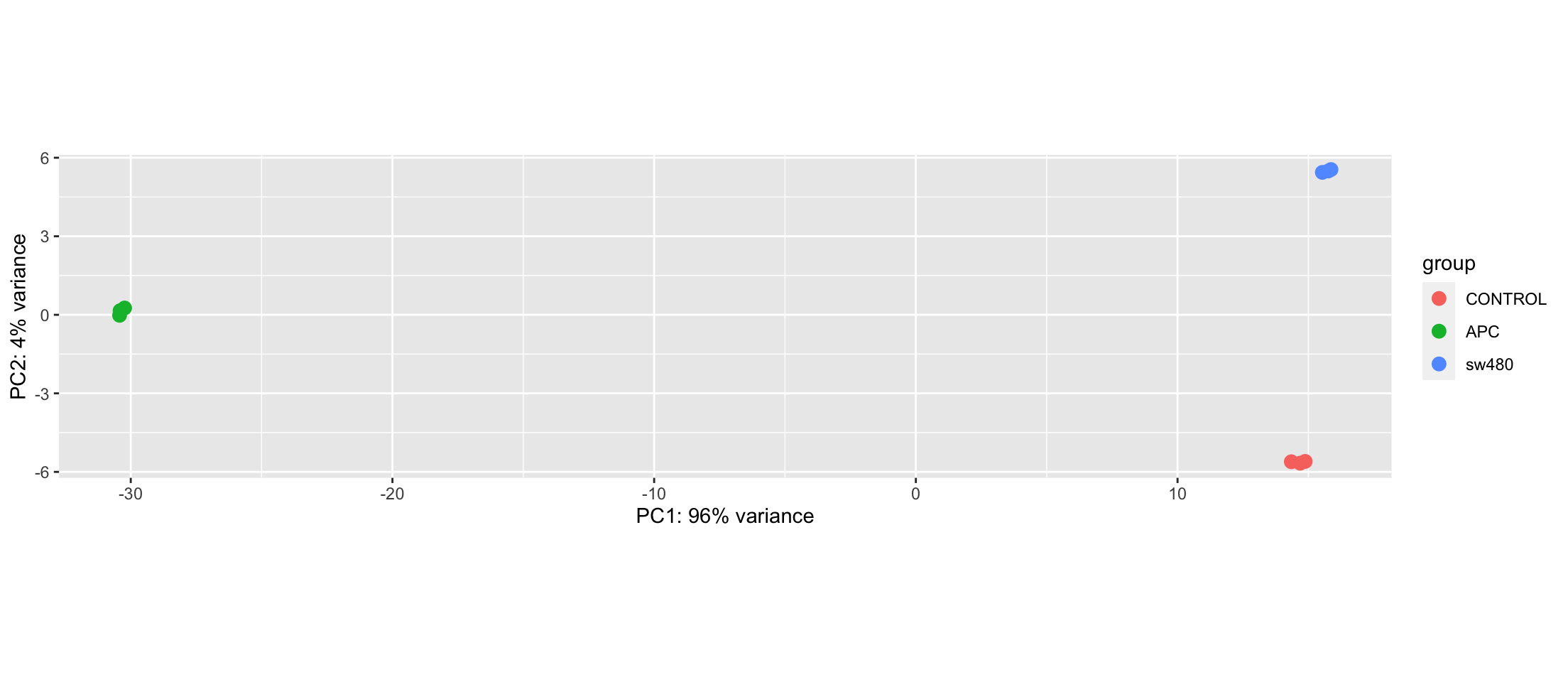# Systems Biology

## Mapping reads to reference genome

There are several tools used to map reads to a genome

• bwa
• bowtie
• bowtie2
• hisat2

Each one has several options, that you can read in their manual

All of them produces SAM/BAM files

## Count Normalization

Using featureCounts (or similar) tool, we transform the SAM file into a matrix of counts

The element $$c_{ij}$$ is the count of reads for gene $$i$$ under library $$j$$

This value depends on

• The gene expression
• The gene length $$l_i$$
• The number of reads in the library $$N_j$$

We care about the first one. The last one depends on technical issues

## First idea: correct library effect

We can normalize the counts by the library size

The library size, i.e. the number of reads in the library, is $N_j = \sum_i c_{ij}$

So the relative number of reads is $\frac{c_{ij}}{N_j}$

## These are small numbers

RNAseq libraries are large. They have millions of read

Thus, each $\frac{c_{ij}}{N_j}$ is a very small number.

We scale them by $$10^6$$ and get Reads Per Million $\frac{c_{ij}⋅10^6}{N_j}$

(same as we scale $$0.000004m$$ to $$4μm$$)

## Larger genes have more reads

If the reads are taken randomly from each mRNA, then longer genes have higher probability of being sequenced

To correct that we divide by the gene length $$l_i$$ $\frac{c_{ij}⋅10^6}{N_j l_i}$

## Average gene length is around 1000bp

Again, this is a small number, so we scale by $$10^3$$ and we get Reads Per Kilo per Million $RPKM_{ij} = \frac{c_{ij}⋅10^9}{N_j l_i}$

## We can change the division order

It is easy to see that $RPKM_{ij} = \frac{c_{ij}⋅10^6}{N_j} \frac{10^3}{l_i} = \frac{c_{ij}⋅10^3}{l_i} \frac{10^6}{N_j}$ so we can divide by the gene length first, and the library size later

When we use paired-end sequencing, we typically get two reads for each fragment

But sometimes we get only one read

This is basically handled by the counting step

FPKM is the same formula as RPKM, but counting fragments instead of reads

## Comparing libraries

We want to compare at least two libraries

The sum of all RPKM in library $$j_1$$ is $\sum_i RPKM_{i{j_1}} = \frac{10^9}{N_{j_1}}\sum_i \frac{c_{ij}}{l_i}$

and, in general, this number is different from the sum for another library $$j_2$$ $\sum_i RPKM_{i{j_1}} ≠ \sum_i RPKM_{i{j_2}}$

That is not good for statistical tests

## Another way to normalize

To avoid this issue, we can normalize by the gene length before calculating totals

Let’s call $$Q_i$$ to the sum of_gene-length-corrected_counts $Q_j = \sum_i \frac{c_{ij}}{l_i}$

Then Transcripts per Million for gene $$i$$ in library $$j$$ is $TPM_{ij}=\frac{c_{ij}}{l_i⋅Q_j}$

## Now

Now we have $\sum_i TPM_{ij}=\frac{1}{Q_j}\sum_i \frac{c_{ij}}{l_i}=1$

## Which one?

• RPKM/FPKM are good for comparing between genes in the same library

• TPM is better for comparing the same gene between libraries

## DESeq2

This tool is based on a different statistical hypothesis

Instead of assuming log-normal distribution, it assumes a negative binomial distribution

Both distributions are similar, but it allows us to see the data from another point of view

In particular, it does not require normalization of counts

## DESeq protocol

library(DESeq2)
head(read.counts)
          sw480_APC_1 sw480_APC_2 sw480_APC_3 sw480_CONTROL_1 sw480_CONTROL_2
NM_000014           1           1           3               1               2
NM_000015           1           0           0               0               2
NM_000016        1038        1126        1098            1285            1037
NM_000017         133         111         103             171             136
NM_000018        8699        8232        8364            8888            8426
NM_000019        2094        2070        1879            2645            2248
sw480_CONTROL_3 sw480.1 sw480.2 sw480.3 cds_length gene_name
NM_000014               2       3       2       2       4653       A2M
NM_000015               1       1       3       1       1317      NAT2
NM_000016            1306    1022    1059    1057       2603     ACADM
NM_000017             174     143     147     125       1917     ACADS
NM_000018            9188   10982   10844   11450       2292    ACADVL
NM_000019            2775    2132    2164    2087       2134     ACAT1

## Describe conditions of each sample

sample_info <- data.frame(
condition=factor(rep(c("APC","CONTROL", "sw480"), each=3),
levels = c("CONTROL", "APC", "sw480")),
sample_info
                condition
sw480_APC_1           APC
sw480_APC_2           APC
sw480_APC_3           APC
sw480_CONTROL_1   CONTROL
sw480_CONTROL_2   CONTROL
sw480_CONTROL_3   CONTROL
sw480.1             sw480
sw480.2             sw480
sw480.3             sw480

## Pack everything in DESeq format

DESeq.ds <- DESeqDataSetFromMatrix( countData = read.counts[,1:9],
colData = sample_info, design = ~ condition)
colData(DESeq.ds)
DataFrame with 9 rows and 1 column
condition
<factor>
sw480_APC_1       APC
sw480_APC_2       APC
sw480_APC_3       APC
sw480_CONTROL_1   CONTROL
sw480_CONTROL_2   CONTROL
sw480_CONTROL_3   CONTROL
sw480.1           sw480
sw480.2           sw480
sw480.3           sw480  
rowData(DESeq.ds) # can be annotation data
DataFrame with 39276 rows and 0 columns

## The data is there

counts(DESeq.ds) |> head()
          sw480_APC_1 sw480_APC_2 sw480_APC_3 sw480_CONTROL_1 sw480_CONTROL_2
NM_000014           1           1           3               1               2
NM_000015           1           0           0               0               2
NM_000016        1038        1126        1098            1285            1037
NM_000017         133         111         103             171             136
NM_000018        8699        8232        8364            8888            8426
NM_000019        2094        2070        1879            2645            2248
sw480_CONTROL_3 sw480.1 sw480.2 sw480.3
NM_000014               2       3       2       2
NM_000015               1       1       3       1
NM_000016            1306    1022    1059    1057
NM_000017             174     143     147     125
NM_000018            9188   10982   10844   11450
NM_000019            2775    2132    2164    2087

## Remove genes without any counts

DESeq.ds <- DESeq.ds[rowSums(counts(DESeq.ds)) > 0, ]

Now we verify that we did not lose any read

all(colSums(counts(DESeq.ds)) == colSums(read.counts[,1:9]))
 TRUE

## Normalization by library

Estimate a factor to correct each library

DESeq.ds <- estimateSizeFactors(DESeq.ds)
sizeFactors(DESeq.ds)
    sw480_APC_1     sw480_APC_2     sw480_APC_3 sw480_CONTROL_1 sw480_CONTROL_2
1.0059487       0.9570850       0.9454599       1.0364710       0.9067061
sw480_CONTROL_3         sw480.1         sw480.2         sw480.3
1.0709679       1.0344438       1.0359450       1.0370205 

## Normalized counts

It is just a factor of scale

counts.sf_normalized <- counts(DESeq.ds, normalized = TRUE)
plot(counts(DESeq.ds)[,1], counts.sf_normalized[,1], pch=".", log="xy")## We can use logarithms

Let’s avoid log2(0) by adding a pseudo-count

log.norm.counts <- log2(counts.sf_normalized + 1)## DESeq does something different

DESeq.rlog <- rlog(DESeq.ds, blind = TRUE)
rlog.norm.counts <- assay(DESeq.rlog)## Comparing conditions## Principal Component Analysis

plotPCA(DESeq.rlog)## Let’s do the statistics

First, gene-wise dispersion estimates across all samples

DESeq.ds <- estimateDispersions(DESeq.ds)
gene-wise dispersion estimates
mean-dispersion relationship
final dispersion estimates

Then a t-test (assuming different variances)

DESeq.ds <- nbinomWaldTest(DESeq.ds)

## Instead of topTable

results(DESeq.ds, independentFiltering = TRUE, alpha = 0.05) |> head()
log2 fold change (MLE): condition sw480 vs CONTROL
Wald test p-value: condition sw480 vs CONTROL
DataFrame with 6 rows and 6 columns
baseMean log2FoldChange     lfcSE      stat      pvalue
<numeric>      <numeric> <numeric> <numeric>   <numeric>
NM_000014    1.889930       0.433904 1.1851497  0.366118 7.14277e-01
NM_000015    0.995613       0.655316 1.6196758  0.404597 6.85774e-01
NM_000016 1111.347112      -0.251112 0.0621873 -4.038000 5.39089e-05
NM_000017  137.250467      -0.254684 0.1441359 -1.766968 7.72335e-02
NM_000018 9407.540815       0.281006 0.0384621  7.306038 2.75134e-13
NM_000019 2224.066114      -0.307231 0.0464157 -6.619114 3.61357e-11
<numeric>
NM_000014          NA
NM_000015          NA
NM_000016 2.67474e-04
NM_000017 1.56518e-01
NM_000018 4.40436e-12
NM_000019 4.61581e-10

## Choosing another condition

results(DESeq.ds, contrast=c("condition", "APC", "CONTROL"),
independentFiltering = TRUE, alpha = 0.05) |> head()
log2 fold change (MLE): condition APC vs CONTROL
Wald test p-value: condition APC vs CONTROL
DataFrame with 6 rows and 6 columns
baseMean log2FoldChange     lfcSE       stat      pvalue
<numeric>      <numeric> <numeric>  <numeric>   <numeric>
NM_000014    1.889930      0.0507329 1.2335629  0.0411271 9.67195e-01
NM_000015    0.995613     -1.4665320 1.8959505 -0.7735076 4.39222e-01
NM_000016 1111.347112     -0.0982781 0.0619814 -1.5856055 1.12829e-01
NM_000017  137.250467     -0.4190118 0.1475355 -2.8400750 4.51029e-03
NM_000018 9407.540815     -0.0189505 0.0387184 -0.4894445 6.24527e-01
NM_000019 2224.066114     -0.2909752 0.0466153 -6.2420551 4.31859e-10
NM_000019 1.76809e-09